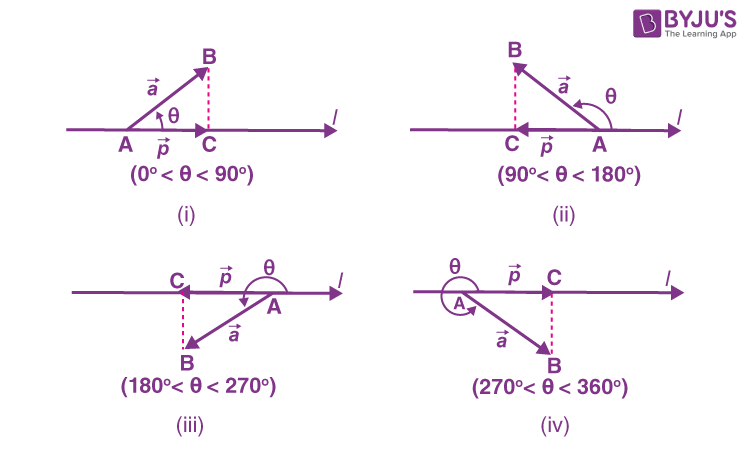# Projection of a Vector on a Line

We know that the vector is a quantity that has both magnitude and direction. There are different types of vectors, such as unit vector, zero vector, collinear vector, equal vector, and so on. The scalar components of a vector are its direction ratios and represent the scalar projections along their respective axes. In this article, we are going to discuss the projection of a vector on a line with many solved examples.

## What is Meant by Projection of a Vector on a Line?

Assume that the vector AB makes an angle θ with the directed line, say “l” in the anti-clock direction as shown in the figure.Thus, the projection of vector AB on the directed line “l” is a vector $$\vec{p}$$ with the magnitude $$|\overline{AB}| |cos \theta |$$, and the direction of $$\vec{p}$$ being the same (or) opposite to that of the directed line “l”. The direction of $$\vec{p}$$ depends upon whether cos θ is positive or negative.

Here, the vector $$\vec{p}$$ is called the projection vector and the magnitude $$|\vec{p}|$$ is called the projection of vector AB on “l”.

In the above figure (i) and (iv), represents the projection of the vector AB along the directed line “l” is the vector AC.

Note:

• If the unit vector $$\hat{p}$$ is along the directed line l, then the projection of $$\vec{a}$$ on the directed line l is given by $$\vec{a}.\hat{p}$$.
• The projection of vector “a” on the vector “b” is given by: $$\vec{a}.\hat{b}$$ or $$\vec{a}.\left ( \frac{\vec{b}}{|\vec{b}|} \right )$$ or $$\frac{1}{|\vec{b}|}(\vec{a}.\vec{b})$$
• If θ = 0, then the projection vector of $$\overline{AB}$$ will be $$\overline{AB}$$.
• If θ = π, then the projection of $$\overline{AB}$$ will be $$\overline{BA}$$.
• If θ = π/2 or θ = 3π/2, then the projection of $$\overline{AB}$$ will be a zero vector.

If α, β and γ be the direction angles of the vector $$\vec{a}=a_{1}\hat{i}+a_{2}\hat{j}+a_{3}\hat{k}$$, then the direction cosines of the given vector is given by the formula,

$$cos \alpha = \frac{\vec{a}.\hat{i}}{|\vec{a}||\hat{i}|} = \frac{a_{1}}{|\vec{a}|}$$ $$cos \beta = \frac{a_{2}}{|\vec{a}|}$$ $$cos \gamma = \frac{a_{3}}{|\vec{a}|}$$

It is observed that $$|\vec{a}|cos\alpha$$, $$|\vec{a}|cos \beta$$, $$|\vec{a}|cos\gamma$$ are the projections of $$\vec{a}$$ along OX, OY and OZ, respectively. It means that the scalar components of a1, a2 and a3 of $$\vec{a}$$, are the projections of $$\vec{a}$$ along x-axis, y-axis and z-axis respectively.

In case $$\vec{a}$$ is a unit vector, then its direction cosines is expressed as:

$$\vec{a}= cos\alpha \hat{i}+ cos\beta \hat{j}+cos\gamma \hat{k}$$

## Projection of a Vector on a Line Examples

Example 1:

Determine the projection of the vector $$\vec{a}= 2\hat{i}+ 3\hat{j}+2\hat{k}$$ on the vector $$\vec{b}= \hat{i}+ 2\hat{j}+\hat{k}$$.

Solution:

Given: $$\vec{a}= 2\hat{i}+ 3\hat{j}+2\hat{k}$$ $$\vec{b}= \hat{i}+ 2\hat{j}+\hat{k}$$

We know that the formula to find the projection of $$\vec{a}$$ on the vector $$\vec{b}$$ is given by:

$$\frac{1}{|\vec{b}|}(\vec{a}.\vec{b})$$

= [2(1) + 3(2) + 2(1)]/√[(1)2+(2)2+(1)3]

= 10/√6

= (5/3)√6.

Hence, the projection of vector $$\vec{a}= 2\hat{i}+ 3\hat{j}+2\hat{k}$$ on the vector $$\vec{b}= \hat{i}+ 2\hat{j}+\hat{k}$$ is (5/3)√6.

Example 2:

Find $$|\vec{x}|$$, if $$\vec{a}$$ is the unit vector and $$(\vec{x}-\vec{a}). (\vec{x}+\vec{a}) = 8$$.

Solution:

Given that: $$\vec{a}$$ is the unit vector. Hence, we can write $$|\vec{a}| = 1$$.

Also, given that $$(\vec{x}-\vec{a}). (\vec{x}+\vec{a}) = 8$$

Now, simplify the above equation,

$$\vec{x}. \vec{x}+\vec{x}.\vec{a}-\vec{a}.\vec{x} – \vec{a}.\vec{a} = 8$$

$$|\vec{x}|^{2} – 1 = 8$$

$$|\vec{x}|^{2} = 8+1$$

$$|\vec{x}|^{2} = 9$$

Therefore, $$|\vec{x}| = \pm 3$$

As, the magnitude of a vector cannot be negative, the value of $$|\vec{x}| = 3$$.

Example 3:

Find the angle between two vectors $$\vec{a}= \hat{i}+\hat{j}-\hat{k}$$ and $$\vec{b}= \hat{i}-\hat{j}+\hat{k}$$.

Solution:

Given: $$\vec{a}= \hat{i}+\hat{j}-\hat{k}$$ $$\vec{b}= \hat{i}-\hat{j}+\hat{k}$$.

The formula to find the angle between two vectors is given by:

$$cos \theta = \frac{\vec{a}.\vec{b}}{|\vec{a}||\vec{b}|}$$

Hence, $$(\vec{a}.\vec{b})= (\hat{i}+\hat{j}-\hat{k}). (\hat{i}-\hat{j}+\hat{k})$$

= 1-1-1

= -1

Therefore, $$(\vec{a}.\vec{b})= -1$$

Now, substituting the value in the formula, we get

Cos θ = -⅓

Hence, the angle between two vectors is θ = cos-1(-⅓).

### Practice Problems

Solve the following problems:

1. Determine the projection of the vector $$\hat{i} – \hat{j}$$ on the vector $$\hat{i} + \hat{j}$$.
2. Determine the projection of vector $$\hat{i} + 3\hat{j}+7\hat{k}$$ on the vector $$7\hat{i} – \hat{j}+ 8\hat{k}$$
3. Compute the angle between two vectors $$\hat{i} – 2\hat{j}+ 3\hat{k}$$ and $$3\hat{i} – 2\hat{j}+ \hat{k}$$.

Keep visiting BYJU’S – The Learning App and download the app to learn all Maths-related concepts by exploring more exciting videos.